# Approximation of functions, measure of

(diff) ← Older revision | Latest revision (diff) | Newer revision → (diff)

A quantitative expression for the error of an approximation. When the discussion is about the approximation of a functionby a function, the measure of approximationis usually defined by the metric in a function space containing bothand. For example, ifandare continuous functions on a segment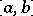, the uniform metric ofis commonly used, i.e. one putsIf continuity of the approximated function is not guaranteed or if the conditions of the problem imply that it is important thatandare close onin an average sense, the integral metric of a space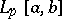may be used, putting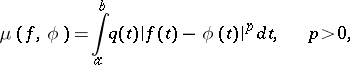whereis a weight function. The case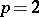is most often used and is most convenient from a practical point of view (cf. Mean-square approximation of a function).

The measure of approximation may take into account only values ofandin discrete points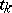,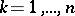, of, e.g.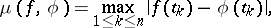where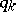are certain positive coefficients.

One defines in an analogous way the measure of approximation of functions in two or more variables.

The measure of approximation of a functionby a family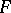of functions is usually taken to be the best approximation:The quantityis usually taken as the measure of approximation of a class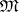of functionsby functionsfrom a certain fixed set. It characterizes the maximal deviation of functions infrom functions inthat are closest to them.

In general, when approximation in an arbitrary metric spaceis considered, the measure of approximationof an elementby an element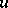(a set) is the distance(or) betweenand(or) in the metric of.

How to Cite This Entry:
Approximation of functions, measure of. Encyclopedia of Mathematics. URL: http://encyclopediaofmath.org/index.php?title=Approximation_of_functions,_measure_of&oldid=18742
This article was adapted from an original article by N.P. KorneichukV.P. Motornyi (originator), which appeared in Encyclopedia of Mathematics - ISBN 1402006098. See original article